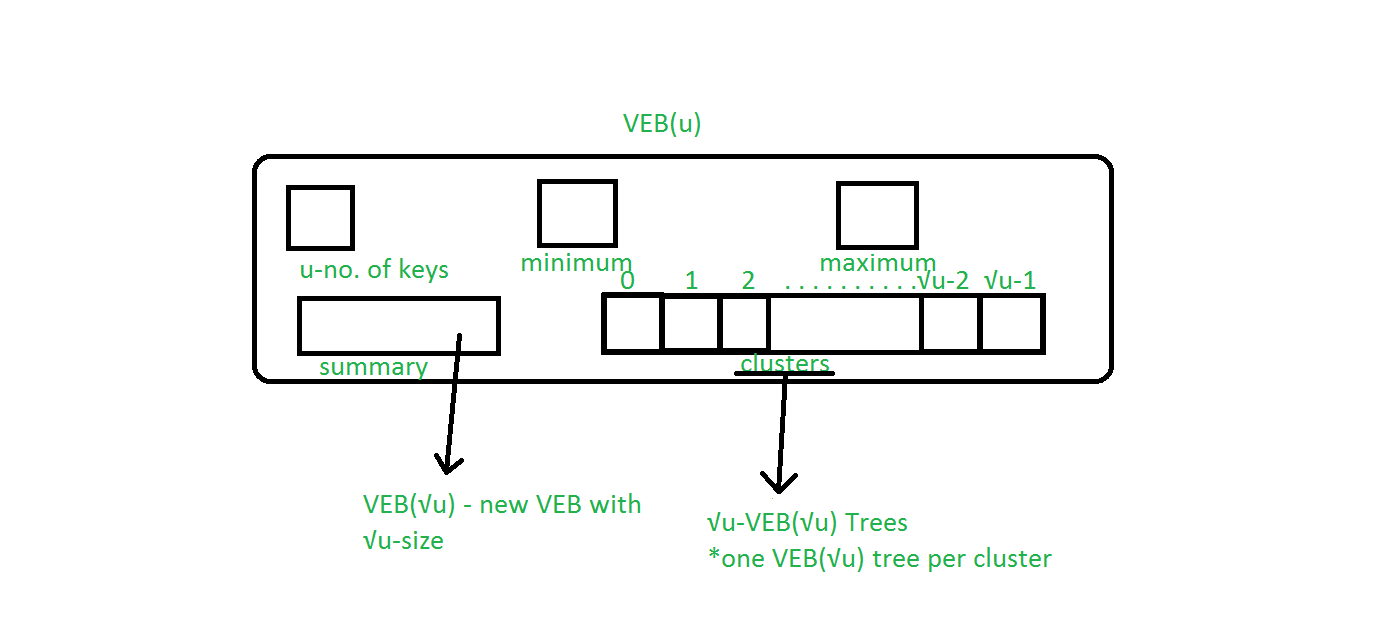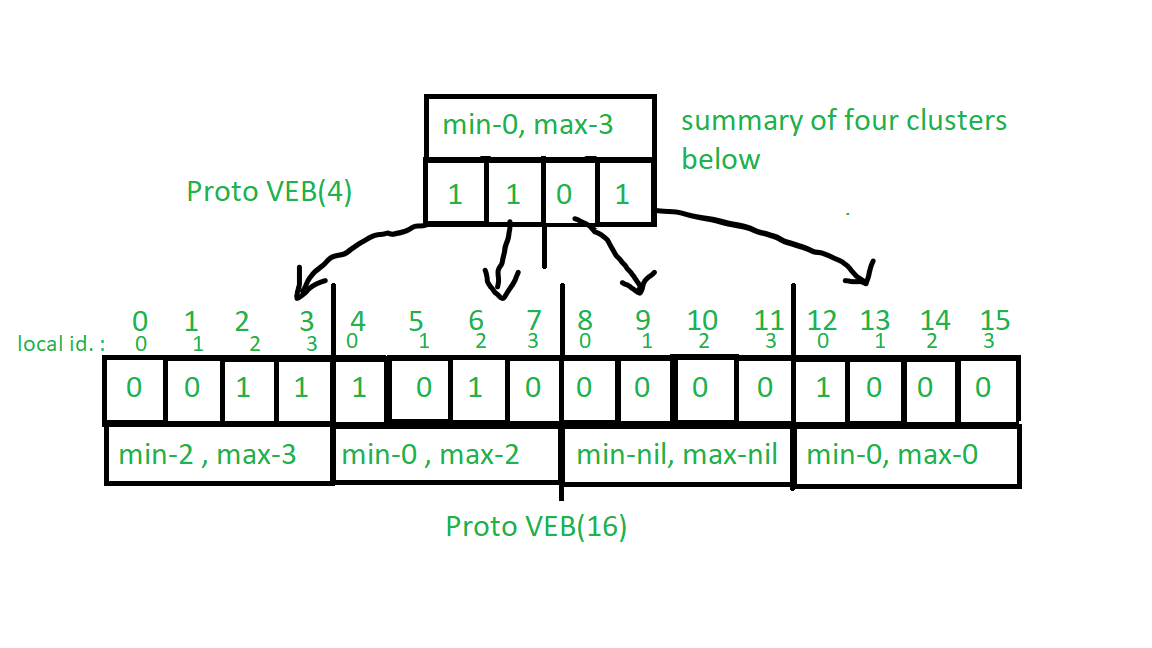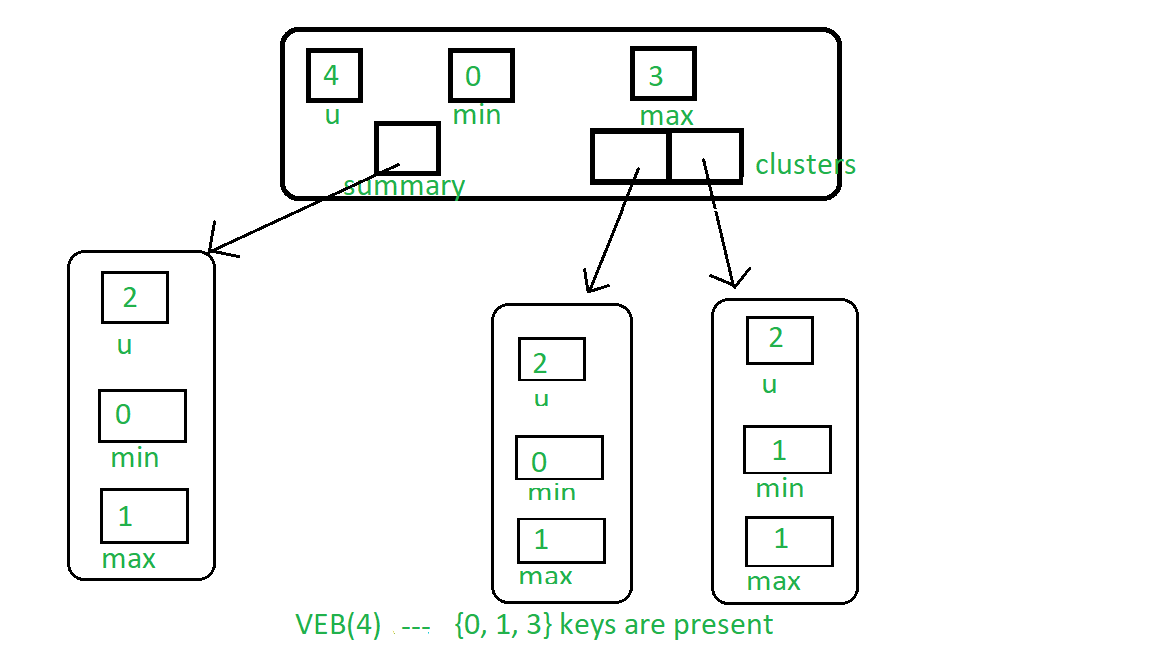Open in App
Not now

# Van Emde Boas Tree | Set 1 | Basics and Construction

• Difficulty Level : Hard
• Last Updated : 12 Aug, 2019

It is highly recommended to fully understand Proto Van Emde Boas Tree.

Van Emde Boas Tree supports search, successor, predecessor, insert and delete operations in O(lglgN) time which is faster than any of related data structures like priority queue, binary search tree, etc. Van Emde Boas Tree works with O(1) time-complexity for minimum and maximum query. Here N is the size of the universe over which tree is defined and lg is log base 2.

Note: Van Emde Boas Data Structure’s key set must be defined over a range of 0 to n(n is positive integer of the form 2k) and it works when duplicate keys are not allowed.

Abbreviations:

1. VEB is an abbreviation of Van Emde Boas tree.
2. VEB() is an abbreviation for VEB containing u number of keys.

Structure of Van Emde Boas Tree:Van Emde Boas Tree is a recursively defined structure.

1. u: Number of keys present in the VEB Tree.
2. Minimum: Contains the minimum key present in the VEB Tree.
3. Maximum: Contains the maximum key present in the VEB Tree.
4. Summary: Points to new VEB() Tree which contains overview of keys present in clusters array.
5. Clusters: An array of sizeeach place in the array points to new VEB() Tree.

See the image below to understand the basics of Van Emde Boas Tree, although it does not represent the actual structure of Van Emde Boas Tree:Basic Understanding of Van Emde Boas Tree:

1. Van Emde Boas Tree is recursively defined structure similar to Proto Van Emde Boas Tree.
2. In Van Emde Boas Tree, Minimum and Maximum queries works in O(1) time as Van Emde Boas Tree stores Minimum and Maximum keys present in the tree structure.
3. Advantages of adding Maximum and Minimum attributes, which help to decrease time complexity:
• If any of Minimum and Maximum value of VEB Tree is empty(NIL or -1 in code) then there is no element present in the Tree.
• If both Minimum and Maximum is equal then only one value is present in the structure.
• If both are present and distinct then two or more elements are present in the Tree.
• We can insert and delete keys by just setting maximum and minimum values as per conditions in constant time( O(1) ) which helps in decreasing recursive call chain: If only one key is present in the VEB then to delete that key we simply set min and max to the nil value. Similarly, if no keys are present then we can insert by just setting min and max to the key we want to insert. These are O(1) operations.
• In successor and predecessor queries, we can take decisions from minimum and maximum values of VEB, which will make our work easier.

In Proto Van Emde Boas Tree the size of universe size is restricted to be of type 22k but in Van Emde Boas Tree, it allows the universe size to be exact power of two. So we need to modify High(x), low(x), generate_index() helper functions used in Proto Van Emde Boas Tree as below.

1. High(x): It will return floor( x/ceil() ), which is basically the cluster index in which the key x is present.

High(x) = floor(x/ceil())
2. Low(x): It will return x mod ceil() which is its position in the cluster.

Low(x) = x % ceil()
3. generate_index(a, b) : It will return position of key from its position in cluster b and its cluster index a.

generate_index(a, b) = a * ceil() + b
4. Construction of Van Emde Boas Tree: Construction of Van Emde Boas Tree is very similar to Proto Van Emde Boas Tree. Difference here is that we are allowing the universe size to be any power of two, so that high(), low(), generate_index() will be different.

To construct, empty VEB: The procedure is the same as Proto VEB just two things minimum and maximum will be added in each VEB. To represent that minimum and maximum is null we will represent it as -1.

Note: In the base case, we just need minimum and maximum values because adding a cluster of size 2 will be redundant after the addition of min and max values.Below is the implementation:

 // C++ implementation of the approach#include using namespace std;  class Van_Emde_Boas {  public:    int universe_size;    int minimum;    int maximum;    Van_Emde_Boas* summary;    vector clusters;      // Function to return cluster numbers    // in which key is present    int high(int x)    {        int div = ceil(sqrt(universe_size));        return x / div;    }      // Function to return position of x in cluster    int low(int x)    {        int mod = ceil(sqrt(universe_size));        return x % mod;    }      // Function to return the index from    // cluster number and position    int generate_index(int x, int y)    {        int ru = ceil(sqrt(universe_size));        return x * ru + y;    }      // Constructor    Van_Emde_Boas(int size)    {        universe_size = size;        minimum = -1;        maximum = -1;          // Base case        if (size <= 2) {            summary = nullptr;            clusters = vector(0, nullptr);        }        else {            int no_clusters = ceil(sqrt(size));              // Assigning VEB(sqrt(u)) to summary            summary = new Van_Emde_Boas(no_clusters);              // Creating array of VEB Tree pointers of size sqrt(u)            clusters = vector(no_clusters, nullptr);              // Assigning VEB(sqrt(u)) to all of its clusters            for (int i = 0; i < no_clusters; i++) {                clusters[i] = new Van_Emde_Boas(ceil(sqrt(size)));            }        }    }};  // Driver codeint main(){    // New Van_Emde_Boas tree with u = 16    Van_Emde_Boas* akp = new Van_Emde_Boas(4);}

My Personal Notes arrow_drop_up